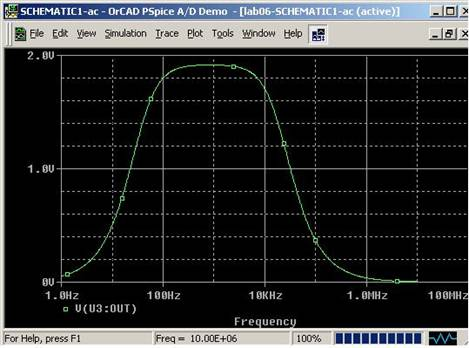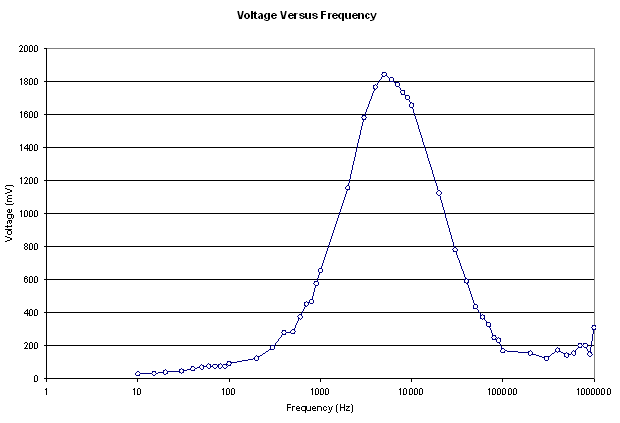Lab 6 Report иC Active Filter

Bill Chun Wai Hung

13 July 2004

A. Describe the Set-up

The setup is shown in figure 1.Figure 1

With a source file of

E_U3         N10100 0 VALUE {LIMIT(V(N09945,0)*1E6,-15V,+15V)}

V_Vi1         N09989 0 DC 0Vdc AC 1Vac

C_C1         N09945 N09877  1u

R_R1         N09989 N09877  4.3k

C_C2         N10100 N09945  1n

R_R2         N10100 N09945  8.25k

B. Desbribe Inputs

R1 = 4.3k ohm

f1 = 37Hz, almost 40Hz

R2 = 8.25k ohm

f2 = 19.3kHz , almost 20kHz

The Op Amp used is LMC64, the kind of op amp that is used in the previous labs.

Stimuli: The Voltage is 1V in amplitude, and the value equals to 2 V peak-to-peak voltage.

With a stimulation of pSpice, the ideal result should be close to the one shown in Figure 2.Figure 2

C. Describe What you Observe

The circuit generated an experimental voltage values shown in Table 1 and Figure 3.

Table 1.

 Frequency (Hz) Voltage (mV) 10 31 15 34 20 41 30 47 40 62 50 72 60 78 70 78 80 78 90 78 100 94 200 125 300 190 400 281 500 285 600 375 700 453 800 469 900 578 1000 656 2000 1156 3000 1582 4000 1766 5000 1844 6000 1812 7000 1781 8000 1734 9000 1703 10000 1656 20000 1125 30000 781 40000 593 50000 437 60000 375 70000 328 80000 250 90000 234 100000 172 200000 156 300000 125 400000 175 500000 144 600000 156 700000 203 800000 203 900000 150 1000000 312Figure 3

D. What you can deduce

The result of the experiment is that. The Experimental Plot of the voltage value resembles the ideal voltage values shown in Figure 2. The peak voltages are both around 1.9V.

What I am planning to do in order to claim my circuit works:

If the input voltage is changed from 1V to 2V, the output voltage would change accordingly. However, the cutoff frequencies will not be change. In other words, the shape of the voltage against frequency plot will still be the same except the whole graph is scaled upward.

The cutoff frequencies (37Hz and 19.3kHz) is not as obvious in the experimental plot. In other words, the voltage change around the cutoff frequencies (37Hz and 19.3kHz) are not as rapid as shown in the ideal plot. This is because the resistors used during the lab are not ideal. A small difference of the resistance from the ideal value changes the frequency response a lot. This is why the voltage rise and drop around the cutoff frequencies are not as rapid as the ideal plot.

This experiment shows the differences between ideal case and the real case. Besides, the purpose of this experiment is to be exposed to active filters. An active filter is incorporating passive filters with op amp. Active filters can change the gain of the op amp to make the output voltage higher when the frequency is in the allowed range (by changing the values of R1 and R2). The gain of the Op-Amp is иCR2/R1. On the other hand, a passive filter is not capable of changing the gain of the output.

Further more, an active filter consist of an op amp, which separate the high-pass and low-pass filter. On the other hand a passive filter is not capable of separating the high-pass and low-pass filter. In other words, the high-pass and low-pass filter in the passive filter would influence each other.

Q3) In the series connection of low pass filter and high pass filter, do the cutoff frequencies change form the original computed f1 and f2?

Yes.

Q4) And if they change, what, do you think, is the reason?

The reason for the change is that originally when the two filters are separated, the filters have separate circuit and separate current. The filters are mutually exclusive in this case. Therefore the filters donбпt influence each other.

However, when the low pass filter and the high pass filter is connected in series. The filters affect each other. When the two filters are connected in series, the filters share the same current, that is the current from the output of the first filter will flow into the input of the second filter. In this case, the filters affect each other, and this cases the cutoff frequencies to be off from f1 and f2.

The solution to prevent the low pass filter and the high pass filter affecting each other is to put an op amp between the low pass filter and the high pass filter.